Generates a procedural tree with

generate_tree(
x = 0,
y = NULL,
z = NULL,
seed = 2000,
midpoint = TRUE,
branch_depth = 6,
branch_scale = c(0.8, 0.9),
branch_angle = c(-30, 30),
branch_angle_vert = seq(-45, 45, by = 5),
branch_split = 2,
branch_prune_prob = 0,
branch_color = "#603000",
leaf_color = "green",
leaf_depth_start = NULL,
leaf_size = 0.2,
leaf_prob = 1,
scale = 1
)

## Arguments

x Default 0. Either the x-coordinate, or if a length-3 vector the x,y, and z coordinates of the base of the tree. Default NULL. The y-coordinate of the base of the tree. Ignored if the x is a length-3 vector. Default NULL. The z-coordinate of the base of the tree. Ignored if the x is a length-3 vector. Default 2. Random seed for generating the tree. Default TRUE. Method of extending branches. If FALSE, it grows directly to the next node. Else, it first extends a midpoint given the previous orientation and grows from there to the end point. Default 6. Number of branch splits to end tree. Default c(0.8,0.9). Default c(-30, 30). Horizontal branching angle from previous branch. Default seq(-45,45, by=5). Vertical branching angle from previous branch. Default 2. Default 0. Default #603000. Default 15. Constant that determines the rate the radius shrinks. Higher values result in less shrinking. Default NULL. Default NULL, automatically set Default 0.01. Default 1. Default 1. Uniformly scale the tree.

## Examples

# \donttest{
library(rayrender)
generate_tree(seed=1) %>%
render_tree()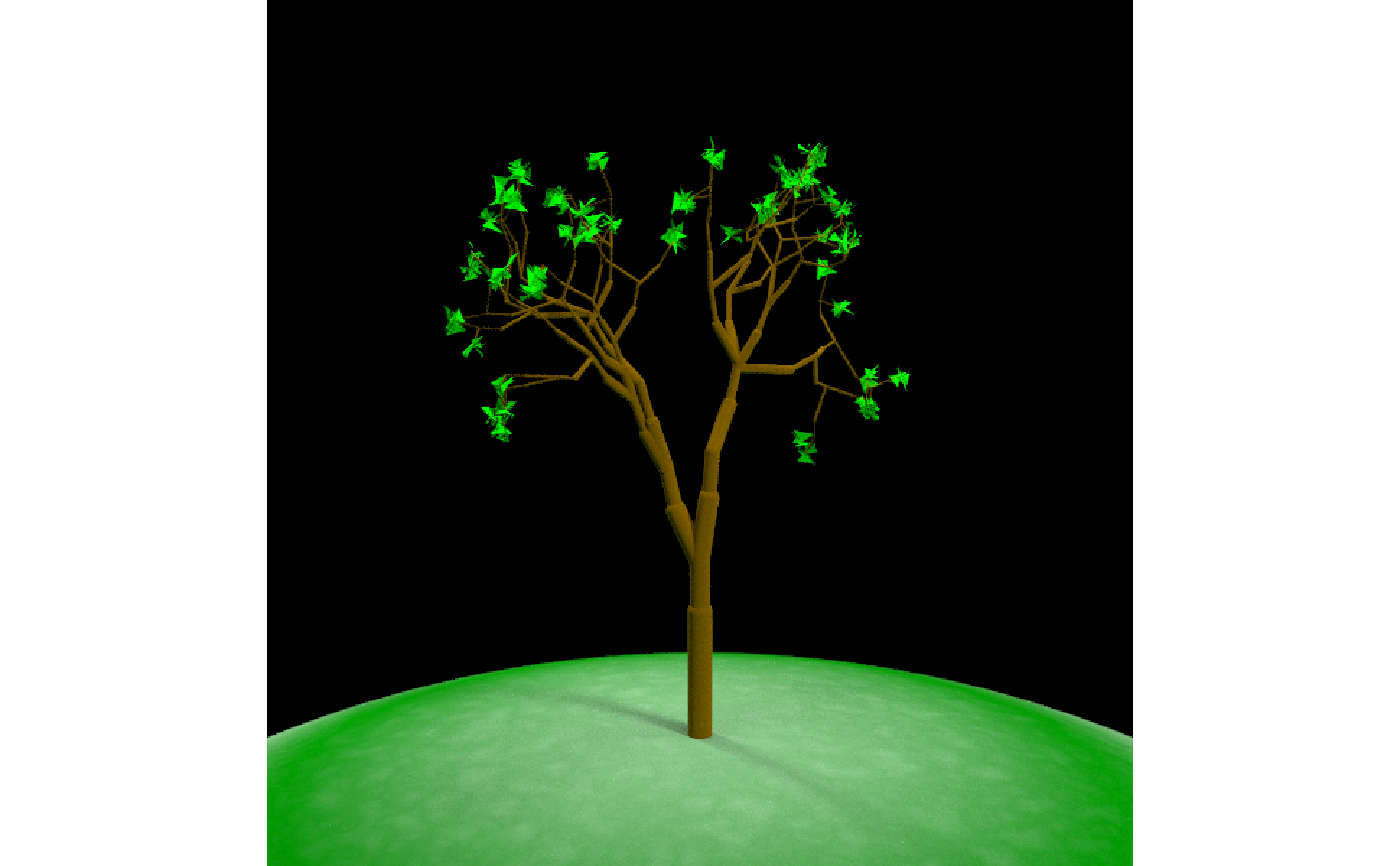#Change the branch angle choices
generate_tree(seed=1,branch_angle_vert = c(-15,15)) %>%
render_tree()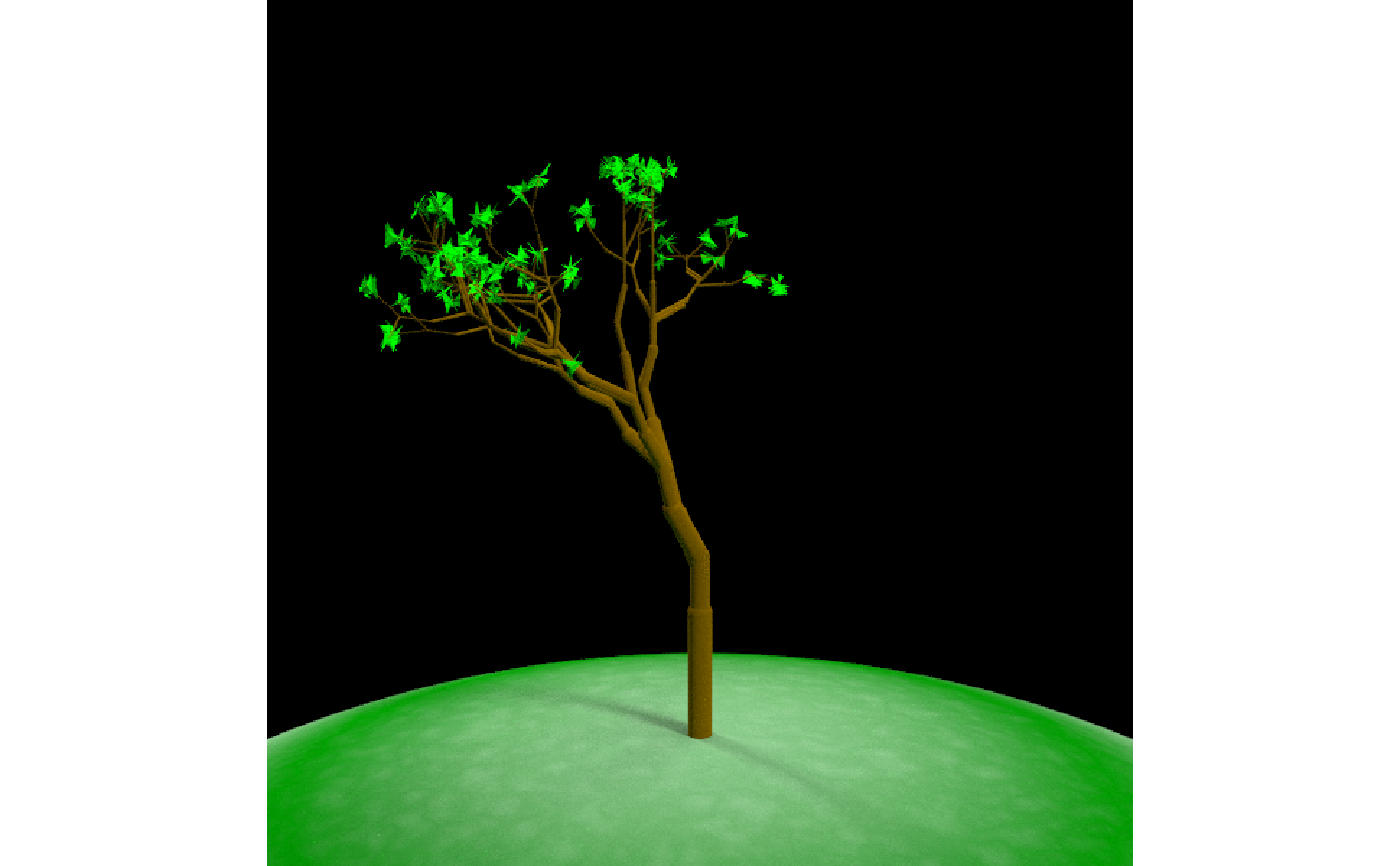#Change the horizontal branch angle choices
generate_tree(seed=6,branch_angle = seq(-90,90,by=10)) %>%
render_tree()#Increase the number of layers
generate_tree(seed=1,branch_depth = 8) %>%
render_tree()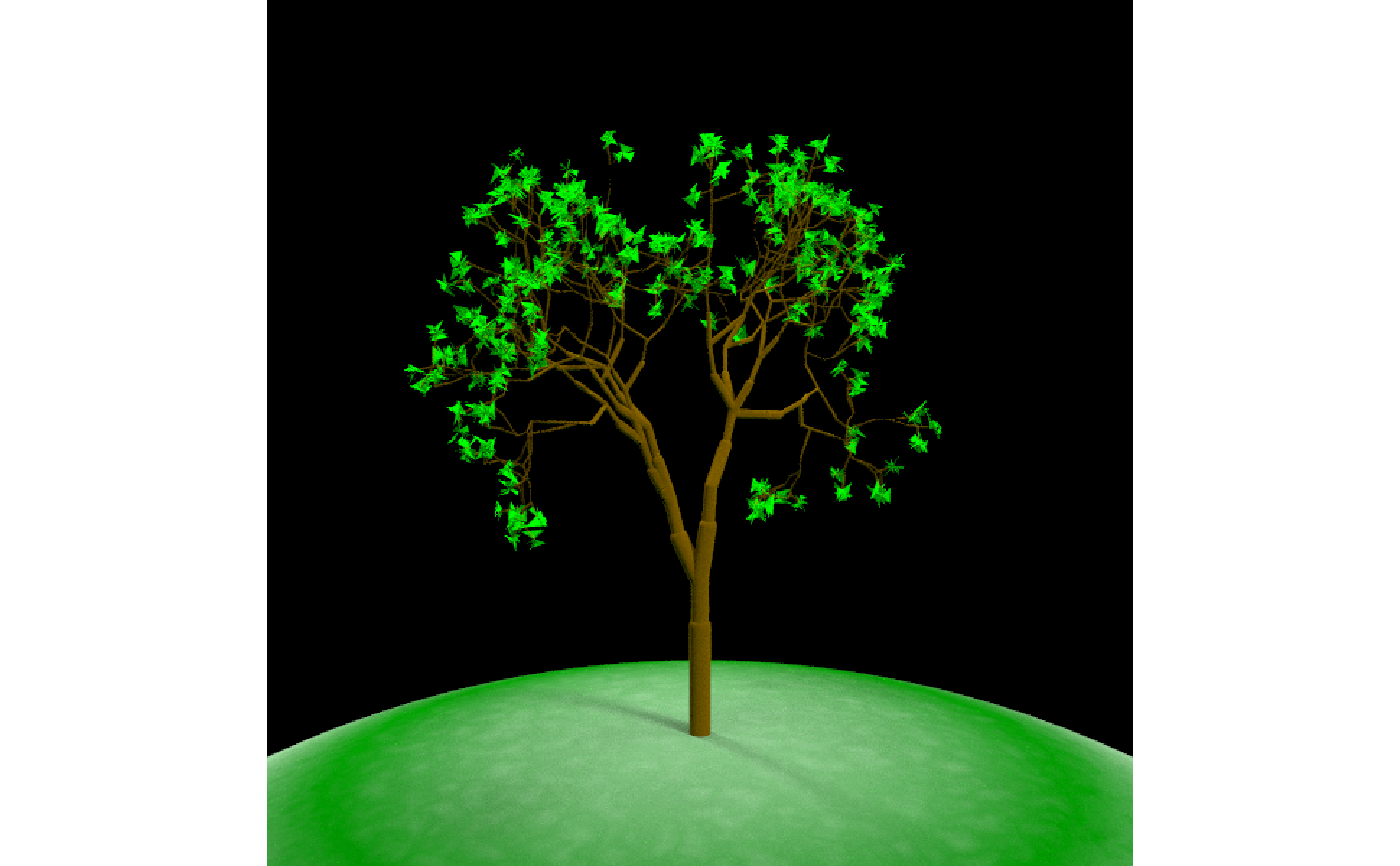#Have the leaves start appearing at branch 6 to fill in the tree
generate_tree(seed=1,branch_depth = 8, leaf_depth_start = 6) %>%
render_tree()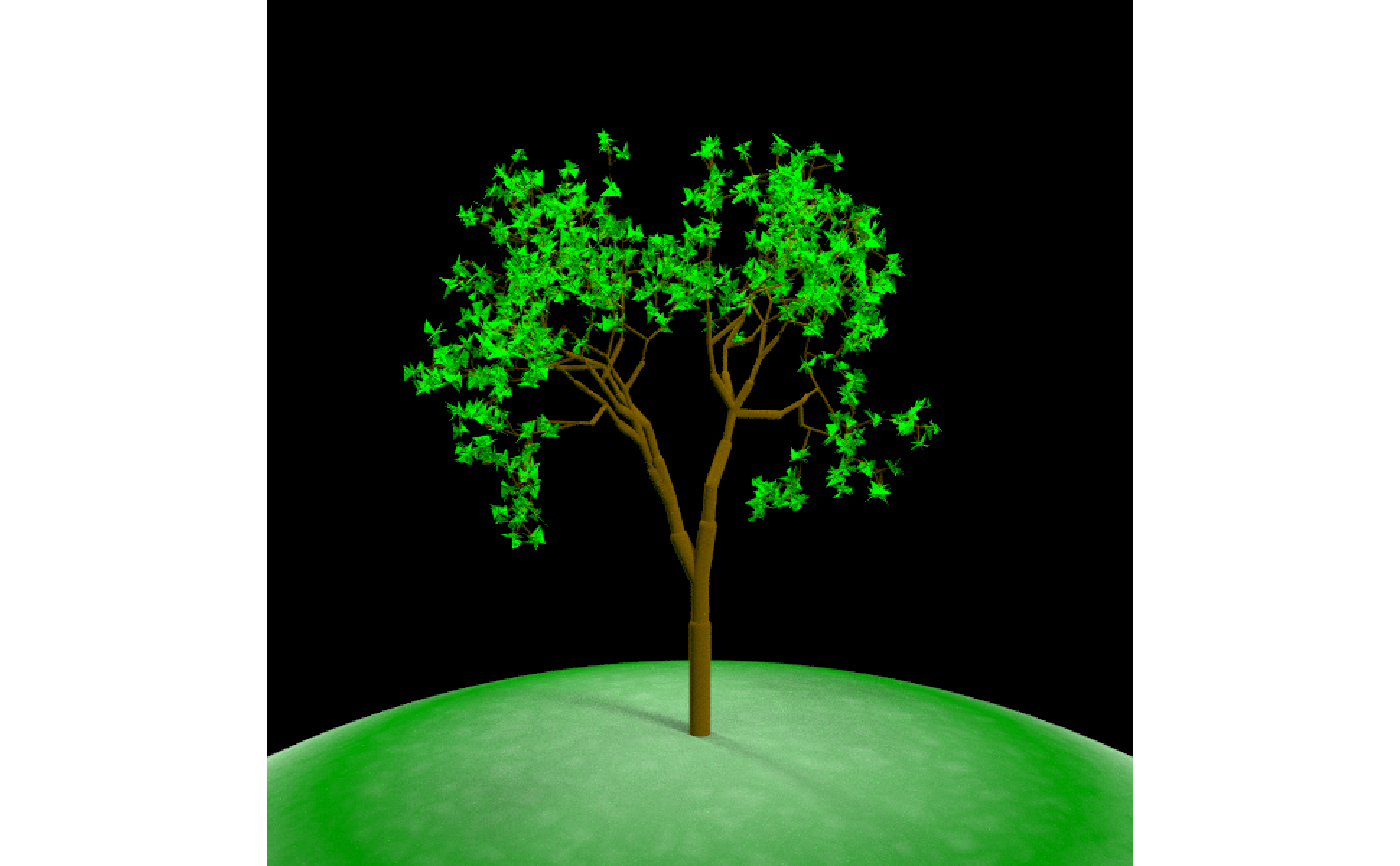#Change the color and seed to get a different structure
generate_tree(seed=2,branch_depth = 6, leaf_depth_start = 4, leaf_color="pink") %>%
render_tree()#Shorten the branches at each junction by random values
generate_tree(seed=2,branch_depth = 6, leaf_depth_start = 4, leaf_color="pink",
branch_scale = c(0.5,0.6)) %>%
render_tree()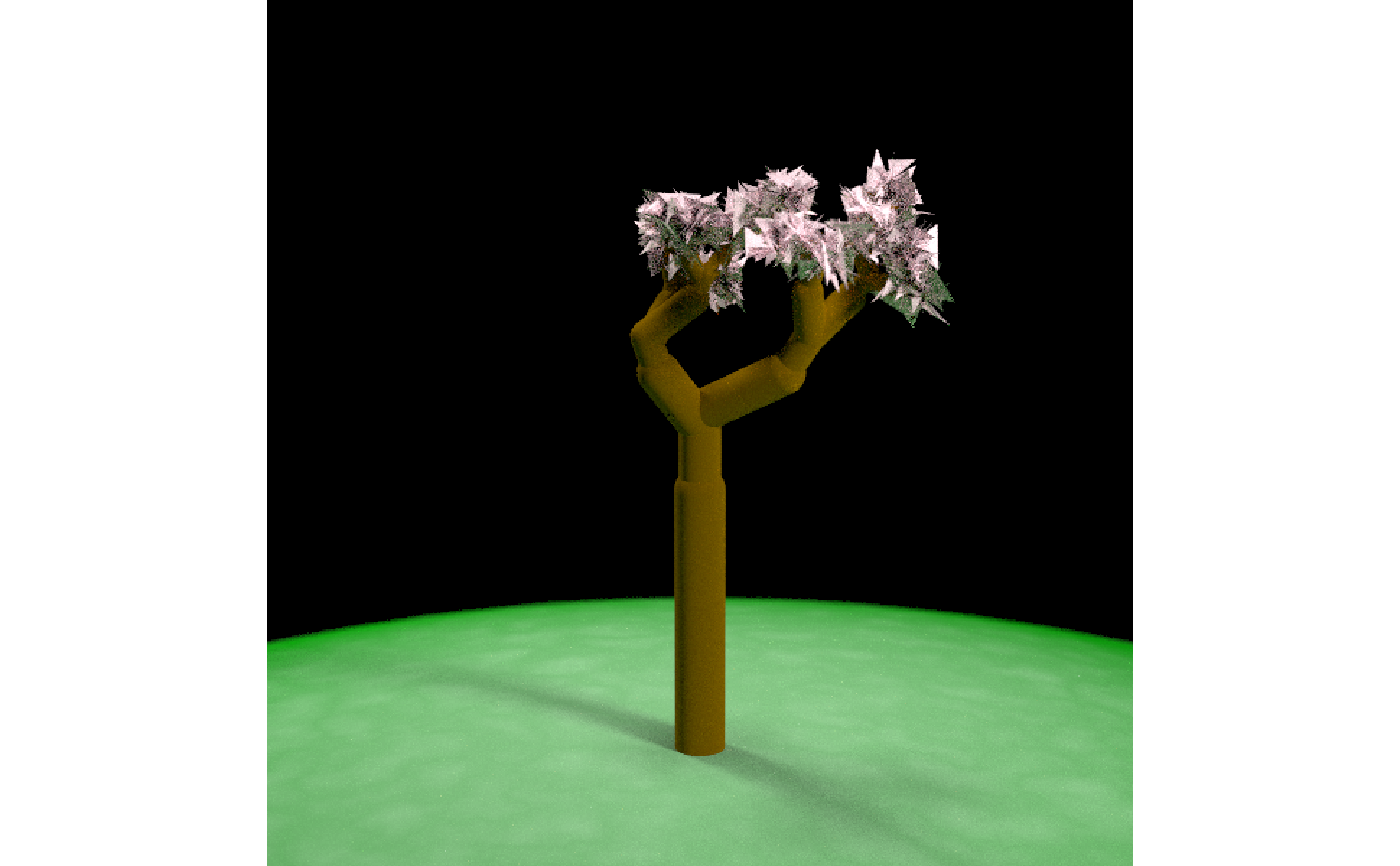#Lengthen the branches at each junction by random values (this results in a wild tree)
generate_tree(seed=2,branch_depth = 6, leaf_depth_start = 4, leaf_color="red",
branch_scale = c(1.1,1.2)) %>%
render_tree()#All angles one sign make the tree lean over, and here we double the size of the leaf
generate_tree(seed=2,branch_depth = 6,
leaf_color="purple", leaf_size=0.4,
branch_angle_vert = c(15,5)) %>%
render_tree()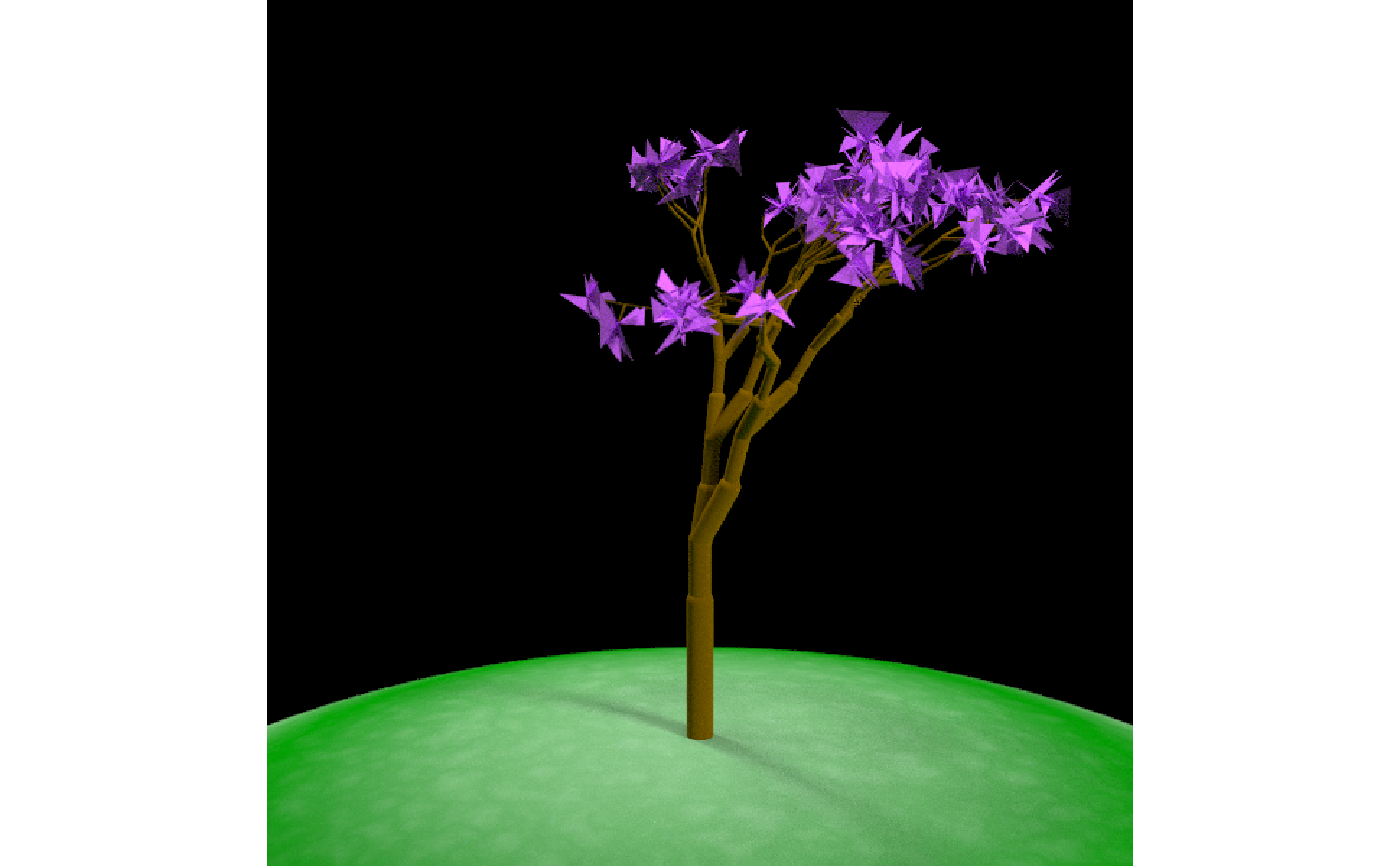#Include a random chance to not grow branches
generate_tree(seed=4,branch_depth = 8, leaf_depth_start = 6, leaf_color="red",
branch_prune_prob = 0.5) %>%
render_tree()# }